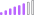cancel
Showing results for
Did you mean:Post Patron

## Create table with measures from a different table as of the selected date in the slicer

Hi,

I want to create a table with measures from a different table as of the selected date in the slicer.

I want to create a table like this where the values in target and percentage are in a different table as measures..  I want the figures to be updated based on the date value selected in the slicer. How do I achieve that?

 Category Target Percentage Week Week Target measure Week % measure MTD MTD Target measure MTD % measure YTD YTD Target measure YTD % measure

My sample data in Table 1

----------------------------

 Date Week Target measure Week % measure MTD Target measure MTD % measure YTD Target measure YTD % measure 01/10/2021 100 0.9 700 0.5 1000 0.75 15/11/2021 50 0.4 200 0.4 1500 0.6 04/12/2021 60 0.3 250 0.3 5000 0.9

and I want to create a new table as shown in the first table for any date value selected in the slicer.

When 01/10/2021 is selected in the slicer, new table should show data as below

 Category Target Percentage Week 100 0.9 MTD 700 0.5 YTD 1000 0.75
1 ACCEPTED SOLUTIONCommunity Support

Hi @bml123 ,

According to your description, here's my solution.

1. Create a new table.

``````Table 2 =
UNION (
ROW ( "Category", "Week" ),
ROW ( "Category", "MTD" ),
ROW ( "Category", "YTD" )
)
``````2. As the calculated column can't change value due to filter, so here should create two measures.

``````Target =
SWITCH (
MAX ( 'Table 2'[Category] ),
"Week",
MAXX (
FILTER ( ALL ( 'Table' ), 'Table'[Date] = SELECTEDVALUE ( 'Table'[Date] ) ),
'Table'[Week Target measure]
),
"MTD",
MAXX (
FILTER ( ALL ( 'Table' ), 'Table'[Date] = SELECTEDVALUE ( 'Table'[Date] ) ),
'Table'[MTD Target measure]
),
"YTD",
MAXX (
FILTER ( ALL ( 'Table' ), 'Table'[Date] = SELECTEDVALUE ( 'Table'[Date] ) ),
'Table'[YTD Target measure]
)
)
``````
``````Percentage =
SWITCH (
MAX ( 'Table 2'[Category] ),
"Week",
MAXX (
FILTER ( ALL ( 'Table' ), 'Table'[Date] = SELECTEDVALUE ( 'Table'[Date] ) ),
'Table'[Week %measure]
),
"MTD",
MAXX (
FILTER ( ALL ( 'Table' ), 'Table'[Date] = SELECTEDVALUE ( 'Table'[Date] ) ),
'Table'[MTD%measure]
),
"YTD",
MAXX (
FILTER ( ALL ( 'Table' ), 'Table'[Date] = SELECTEDVALUE ( 'Table'[Date] ) ),
'Table'[YTD % measure]
)
)
``````

Get the expected result.I attach my sample below for reference.

Best Regards,
Community Support Team _ kalyj

If this post helps, then please consider Accept it as the solution to help the other members find it more quickly.

5 REPLIES 5Community Support

Hi @bml123 ,

According to your description, here's my solution.

1. Create a new table.

``````Table 2 =
UNION (
ROW ( "Category", "Week" ),
ROW ( "Category", "MTD" ),
ROW ( "Category", "YTD" )
)
``````2. As the calculated column can't change value due to filter, so here should create two measures.

``````Target =
SWITCH (
MAX ( 'Table 2'[Category] ),
"Week",
MAXX (
FILTER ( ALL ( 'Table' ), 'Table'[Date] = SELECTEDVALUE ( 'Table'[Date] ) ),
'Table'[Week Target measure]
),
"MTD",
MAXX (
FILTER ( ALL ( 'Table' ), 'Table'[Date] = SELECTEDVALUE ( 'Table'[Date] ) ),
'Table'[MTD Target measure]
),
"YTD",
MAXX (
FILTER ( ALL ( 'Table' ), 'Table'[Date] = SELECTEDVALUE ( 'Table'[Date] ) ),
'Table'[YTD Target measure]
)
)
``````
``````Percentage =
SWITCH (
MAX ( 'Table 2'[Category] ),
"Week",
MAXX (
FILTER ( ALL ( 'Table' ), 'Table'[Date] = SELECTEDVALUE ( 'Table'[Date] ) ),
'Table'[Week %measure]
),
"MTD",
MAXX (
FILTER ( ALL ( 'Table' ), 'Table'[Date] = SELECTEDVALUE ( 'Table'[Date] ) ),
'Table'[MTD%measure]
),
"YTD",
MAXX (
FILTER ( ALL ( 'Table' ), 'Table'[Date] = SELECTEDVALUE ( 'Table'[Date] ) ),
'Table'[YTD % measure]
)
)
``````

Get the expected result.I attach my sample below for reference.

Best Regards,
Community Support Team _ kalyj

If this post helps, then please consider Accept it as the solution to help the other members find it more quickly.Post Patron

It worked perfectly fine.  Thank you very muchSuper User

@bml123 , if your source table is like that, you can try this power query code. use it blank query and check the steps

``````let
Source = Table.FromRows(Json.Document(Binary.Decompress(Binary.FromText("RY3BDcAgDAN3yRs1dkpAnQWx/xrQBsrHOsc6pTUBlVCDUZIQmInrmVkXe9yjVJeemtCV3JLHlGcaDtNXKZ+CrLStlFjuV/HDjv9/7wM=", BinaryEncoding.Base64), Compression.Deflate)), let _t = ((type nullable text) meta [Serialized.Text = true]) in type table [Date = _t, #"Week Target measure" = _t, #"Week % measure" = _t, #"MTD Target measure" = _t, #"MTD % measure" = _t, #"YTD Target measure" = _t, #"YTD % measure" = _t]),
#"Changed Type" = Table.TransformColumnTypes(Source,{{"Date", type text}, {"Week Target measure", Int64.Type}, {"Week % measure", type number}, {"MTD Target measure", Int64.Type}, {"MTD % measure", type number}, {"YTD Target measure", Int64.Type}, {"YTD % measure", type number}}),
#"Unpivoted Other Columns" = Table.UnpivotOtherColumns(#"Changed Type", {"Date"}, "Attribute", "Value"),
#"Split Column by Delimiter" = Table.SplitColumn(#"Unpivoted Other Columns", "Attribute", Splitter.SplitTextByDelimiter(" ", QuoteStyle.Csv), {"Attribute.1", "Attribute.2", "Attribute.3"}),
#"Changed Type1" = Table.TransformColumnTypes(#"Split Column by Delimiter",{{"Attribute.1", type text}, {"Attribute.2", type text}, {"Attribute.3", type text}}),
#"Replaced Value" = Table.ReplaceValue(#"Changed Type1","%","Percent",Replacer.ReplaceText,{"Attribute.2"}),
#"Pivoted Column" = Table.Pivot(#"Replaced Value", List.Distinct(#"Replaced Value"[Attribute.2]), "Attribute.2", "Value")
in
#"Pivoted Column"``````Super User

@bml123
Can you share sample data and sample output in table format? Or a sample pbix after removing sensitive data.

but calculation group can help refer this

Calculation Groups- Measure Slicer, Measure Header Grouping, Measure to dimension conversion. Complex Table display: https://youtu.be/qMNv67P8Go0Post Patron

I have posted sample data and the expected output above.  Please can you let me know how to achieve that? Thank you.Announcements#### Exclusive opportunity for Women!

Join us for a free, hands-on Microsoft workshop led by women trainers for women where you will learn how to build a Dashboard in a Day!#### Power Platform Conference-Power BI and Fabric Sessions

Join us Oct 1 - 6 in Las Vegas for the Microsoft Power Platform Conference.Top Solution Authors
Top Kudoed Authors
Users online (2,325)